## 1. Introduction

This Project is about making an educated guess to derive an unknown password based on hacked password lists (Google: “RockYou”). In this section we will introduce the mathematical framework to handle passwords and develop a simple brute force approach based on very unrealistic Assumptions. During this Project these Assumptions will then be weaken to  derive in the end a clever method to guess passwords.

### 1.1. Formulation

Letbe a password and the random variables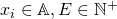the corresponding letters and password length.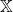is the space of all passwords andcorresponds to the used alphabet, for example if we allow only for lower case letters then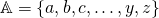. As usual this forms a discrete probability space given by.

## 2. First Attempts

### 2.1 A first naive approach

Letbe the true password. The idea of a brute force search, where every possible password is successive tested, is then to assume that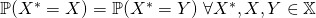. This means that every possible password is equipropable and the testing order is thus irrelevant. It is obvious that this Assumption is not true in general.

### 2.2 A very simple Educated Guess Procedure

In the next step we will replace this assumption by some less strong assumptions:

1. E is distributed with distribution.
2.are independent and identically distributed random variables with distribution.
3.andare independent.

Under the given Assumptions the best way to “guess” the password is given by the following procedure. Sinceandare independent we can apply a two step procedure where we first draw some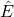using.Then eachis independently drawn using. Sinceandare unknown they have to be estimated from hacked passwords list which we will denote as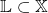.

#### 2.2.1 Checking the password security

Under the given Assumptions it is also possible to estimate the “safeness” of given password. Mathematically this means 1 minus the probability that a certain password is “guessed” by the procedure. The first part, the probability that the “correct” password length is “guessed” is simply given by. The second part can be understood asurn draws with replacement of an urn containing the elements ofwhere the probability to draw a certain elementis given by. Thus. Accordingly by Bayes’ theorem:as security index.

#### 2.2.2 Density Estimators

A very simple estimatoris based on counting the length of all passwords in. The corresponding estimator is then given byand accordingly.